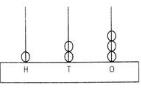Courses

# Test: Tens And Ones - 2

## 20 Questions MCQ Test Mathematics (Maths) for Class 2 | Test: Tens And Ones - 2

Description
This mock test of Test: Tens And Ones - 2 for Class 2 helps you for every Class 2 entrance exam. This contains 20 Multiple Choice Questions for Class 2 Test: Tens And Ones - 2 (mcq) to study with solutions a complete question bank. The solved questions answers in this Test: Tens And Ones - 2 quiz give you a good mix of easy questions and tough questions. Class 2 students definitely take this Test: Tens And Ones - 2 exercise for a better result in the exam. You can find other Test: Tens And Ones - 2 extra questions, long questions & short questions for Class 2 on EduRev as well by searching above.
QUESTION: 1

Solution:
QUESTION: 2

Solution:
QUESTION: 3

### What is the place value of 8 in 79683?

Solution:
QUESTION: 4

Find the place of 0 in 67230.

Solution:
QUESTION: 5

The face value of 7 in 5674 is:

Solution:
QUESTION: 6

Identify the digit at hundreds in 56783.

Solution:
QUESTION: 7

What is the place value of digit 4 in 673425.

Solution:
QUESTION: 8

Find the number if digit at unit place of a number is successor of 7 and digit at tens place is successor of 5.

Solution:
QUESTION: 9

How much should be added with 9 to get the number which has units 0, tens 0, hundreds 1?

Solution:
QUESTION: 10

How much least number should be subtracted from 56 to get the resulting number which has digit 5 at units place?

Solution:
QUESTION: 11

What should be subtracted from 100 to get the resulting number which has two equal digits each of one digit greatest number.

Solution:
QUESTION: 12

The digit at unit place is 2 greater than the digit at tens place then which one of the following may be the possible number?

Solution:
QUESTION: 13

Which one of the following is more than 10 tens?

Solution:
QUESTION: 14

Write the number shown in following abacus?Solution:
QUESTION: 15

Smallest three digit number is:

Solution:
QUESTION: 16

Which one of the following is correct about the places of digits of 7654.

Solution:
QUESTION: 17

Which one of the following numbers is obtained if units > 5, tens < 10 and hundreds is = 3

Solution:
QUESTION: 18

Identify the digit which is at thousands place in 56834.

Solution:
QUESTION: 19

499 comes just before:

Solution:
QUESTION: 20

Which one of the following options is correct to fill in the blank?
224 = 2 hundreds + 2 tens + _______ones

Solution: